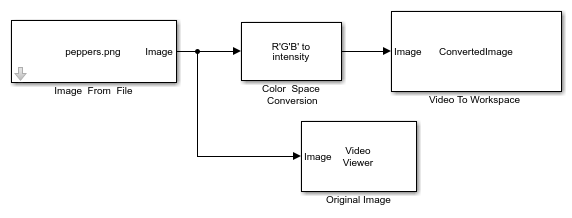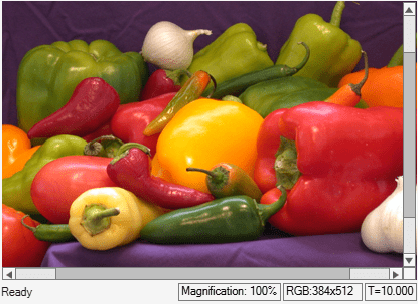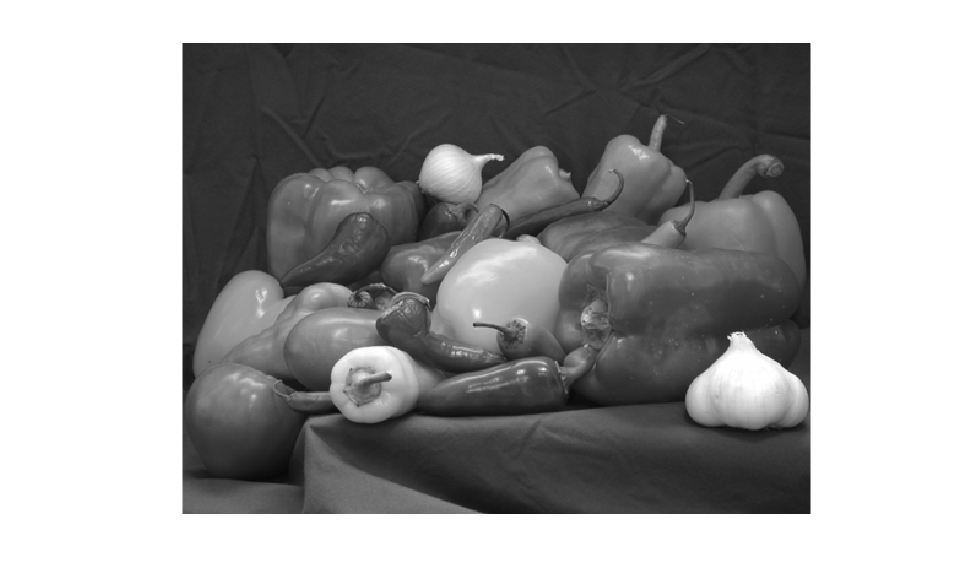# Export Image to MATLAB Workspace

This model shows how to export an image from Simulink to MATLAB workspace by using the `Video` `To` `Workspace` block.

### Example Model

This model takes a color image as the input, converts it into a gray scale image and exports the converted image to MATLAB workspace.

```modelname='ex_blkvideotoworkspace.slx'; open_system(modelname); ```To convert the original image into gray scale, set the `Conversion` parameter of `Color` `Space` `Conversion` block to `R'G'B` `to` `intensity`. The original image is of size 384-by-512-by-3 and the gray scale image output from the `Color` `Space` `Conversion` block is of size 384-by-512.

Export the converted image to MATLAB workspace as a variable named `ConvertedImage` using the `Video` `To` `Workspace` block. You can display the original image using the `Video` `Viewer` block.

### Simulate and Display Results

```sim(modelname); ```The `Video` `To` `Workspace` block exports the converted image as a video with two identical frames and is of size 384-by-512-by-2. Use the `imshow` function to display the first frame in the video.

```imshow(ConvertedImage(:,:,1)) ```